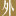# Bund Center Investment Ltd SGX:BTEBund Center Investment Ltd
SGX:BTE
Price: 0.425 SGD
Updated:

## DCF Value

This DCF valuation model was created byAlpha Spread and was last updated on Nov 25, 2023.

Estimated DCF Value of oneBTE stock is 0.365 SGD. Compared to the current market price of 0.425 SGD, the stock is Overvalued by 14%.

DCF Valuation FAQ:
BTE DCF Value
Base Case
0.365 SGD
Overvaluation 14%
DCF Value
Price0.365
DCF Value
Bund Center Investment Ltd Competitors:
DCF Valuation

Revenue
91.6m 96.3m
Net Income
16m 18.1m

## Present Value Calculation

The first step in the DCF calculation process is to estimate the company's future free cash flow. Once free cash flow is forecasted, it is discounted at a risk-appropriate discount rate. The resulting value is the present value of the company's free cash flow. You can change any model inputs below.

Available Valuation Models
1 valuation model availableUpdated on Nov 25, 2023
Default Valuation
Other investors haven't shared any valuation models for Bund Center Investment Ltd.
Model Settings
Operating Model
Discount Rate
6.43%
Forecast Period
5 Years
Terminal Growth
0%
Discount Rate
6.43%
Terminal Growth
0%
Growth Period
5 Years

### DCF Model Base Case ScenarioDCF Model
Currency: SGD
Thousands

To view the process of deriving the DCF Value of one share from the estimated Present Value, see the DCF Value Calculation block.

## DCF Value Calculation

Depending on which type of operating model is chosen (equity or whole firm valuation model), the resulting value is either the value of equity or the value of the entire firm. In the case of the latter, to move from the value of the firm to the value of equity, liabilities are subtracted and assets are added.

### Capital Structure From Present Value to DCF ValuePresent Value 277m SGD Equity Value 277m SGD / Shares Outstanding 759m BTE DCF Value 0.365 SGD
Overvalued by 14%

To view the process of calculating the Present Value of Bund Center Investment Ltd' future free cash flow, see the Present Value Calculation block.

Estimated DCF Value of oneBTE stock is 0.365 SGD. Compared to the current market price of 0.425 SGD, the stock is Overvalued by 14%.
1. Present Value Calculation. Using the DCF Operating Model we projectBund Center Investment Ltd's future free cash flow and discount it at a selected discount rate to calculate its Present Value (277m SGD).
2. DCF Value Calculation. We use the company's capital structure to calculate the total Equity Value based on the previously computed Present Value of the free cash flow. Dividing the Equity Value by the number of shares outstanding gives us the DCF Value of 0.365 SGD per oneBTE share.